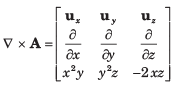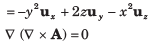Courses

# Vector Analysis - MCQ Test

## 20 Questions MCQ Test RRB JE for Electrical Engineering | Vector Analysis - MCQ Test

Description
This mock test of Vector Analysis - MCQ Test for Railways helps you for every Railways entrance exam. This contains 20 Multiple Choice Questions for Railways Vector Analysis - MCQ Test (mcq) to study with solutions a complete question bank. The solved questions answers in this Vector Analysis - MCQ Test quiz give you a good mix of easy questions and tough questions. Railways students definitely take this Vector Analysis - MCQ Test exercise for a better result in the exam. You can find other Vector Analysis - MCQ Test extra questions, long questions & short questions for Railways on EduRev as well by searching above.
QUESTION: 1

### The two vector are RAM = 20ux + 18uy - 10uz and RAN = -10ux + 8uy + 15uz. The u of the triangle that bisects the interior angle at A is

Solution:

The non-unit vector in the required direction is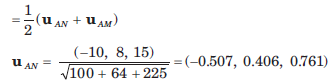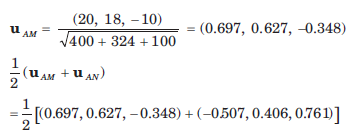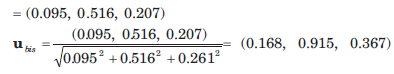QUESTION: 2

### Two points in cylindrical coordinates are A(ρ = 5, Ø = 700 , z = -3) and B(ρ = 2, Ø = 300 , z = 1) A unit vector at A towards B is

Solution:

In cartesian coordinates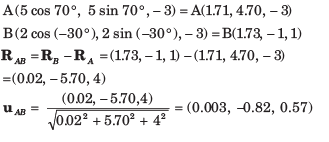QUESTION: 3

### A field in cartesian form is given as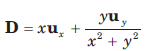In cylindrical form it will be

Solution: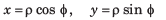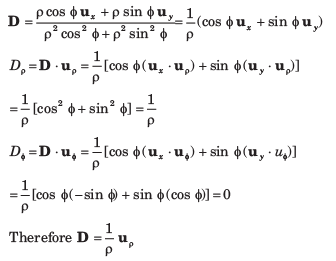QUESTION: 4

The surface ρ = 2, ρ = 4,  Ø = 1000  ,Ø = 1300 ,z = 3  and z = 4.5 define a closed surface. The enclosed volume is

Solution: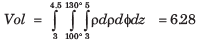QUESTION: 5

The surface ρ = 2, ρ = 4,  Ø = 450  ,Ø = 1350 ,z = 3  and z = 4 define a closed surface. The total area of the enclosing surface is

Solution:

Area is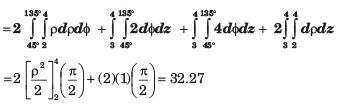QUESTION: 6

The surface ρ = 3, ρ = 5,  Ø = 1000  ,Ø = 1300 ,z = 3  and z = 4.5 define a closed surface. The length of the longest straight line that lies entirely within the volume is

Solution: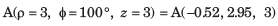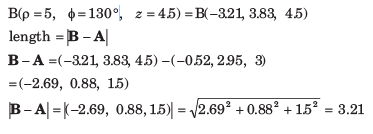QUESTION: 7

A vector field H is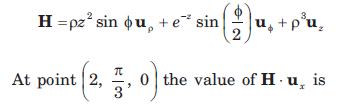Solution: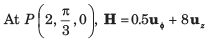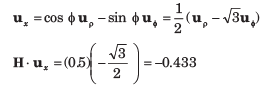QUESTION: 8

A vector is A = yux +(x+z)uy  .At point P(-2, 6, 3)  A in cylindrical coordinate is

Solution: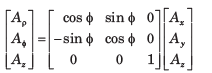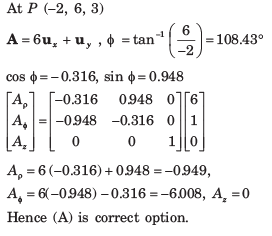QUESTION: 9

The directional derivative of function φ = xy + yz + zx at point P(3, -3, -3) in the directiontoward point Q(4, -1, -1) is

Solution: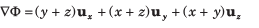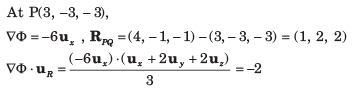QUESTION: 10

The temperature in a auditorium is given by T = 2x2 +y2 - 2z2 . A mosquito located at (2, 2, 1) in the auditorium desires to fly in such a direction that it will get warm as soon as possible. The direction, in that it must fly is

Solution: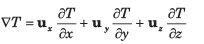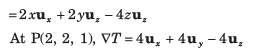QUESTION: 11

The angle between the normal to the surface x2y + z = 3 and x ln z - y2 = -4 at the point of intersection (-1, 2, 1) is

Solution: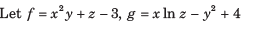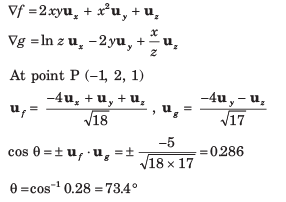QUESTION: 12

The divergence of vector A = yzux + 4xyuy + yuz at point P(1, -2, 3) is

Solution: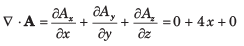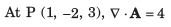QUESTION: 13

The divergence of the vector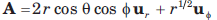at point P(1, 300 , 600 ) is

Solution: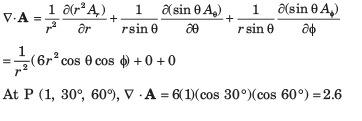QUESTION: 14

Which of the quantities is nearest to the value of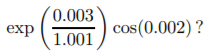Solution:
QUESTION: 15

The flux of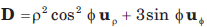over the closed surface of the cylinder 0 ≤ z ≤ 3 , ρ = 3 is

Solution:

By divergence theorem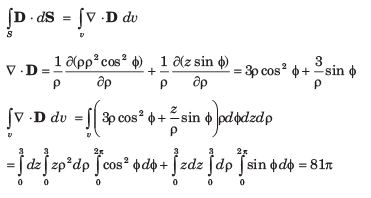QUESTION: 16

The curl of vector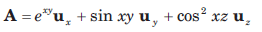is

Solution: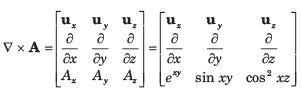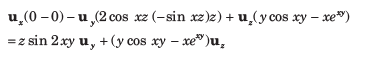QUESTION: 17

The curl of vector field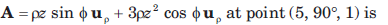Solution: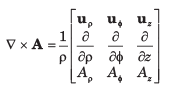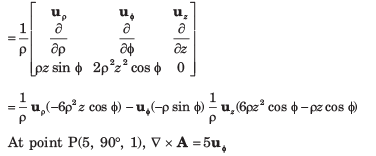QUESTION: 18

The curl of vector field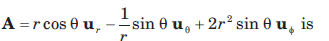Solution: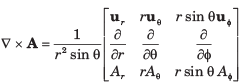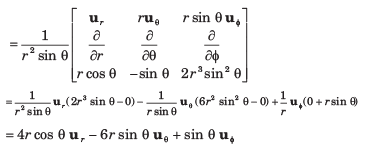QUESTION: 19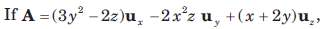the value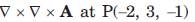is

Solution: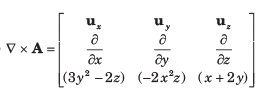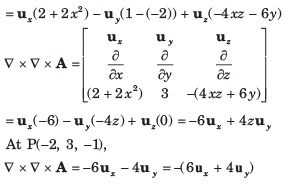QUESTION: 20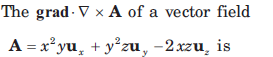Solution: